Most Affordable JEE | NEET | 8,9,10 Preparation by Kota's Top IITian Doctor Faculties

# What is Motion of bodies connected by strings ?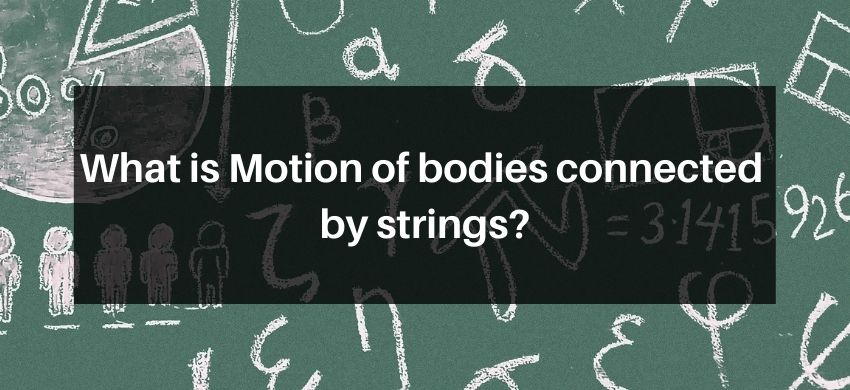## Motion of bodies connected by Strings

Two bodies: Let us consider the case of two bodies of masses $m_{1}$ and $m_{2}$ connected by a thread and placed on a smooth horizontal surface as shown in fig. A force $F$ is applied on the body of mass $m_{2}$ in forward direction as shown. Our aim is to consider the acceleration of the system and the tension $\mathrm{T}$ in the thread. The forces acting separately on two bodies are also shown in the figure: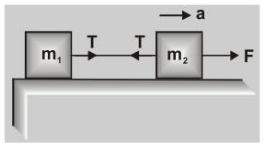From fig. $\quad \mathrm{T}=\mathrm{m}_{1} \mathrm{a}$             .....(1)

$\mathrm{F}-\mathrm{T}=\mathrm{m}_{2} \mathrm{a}$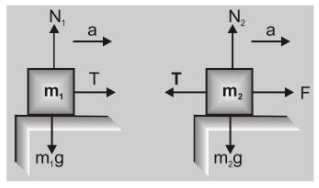$F=\left(m_{1}+m_{2}\right) a$

or $a=\frac{F}{m_{1}+m_{2}}$   .....(3)

The acceleration of the system can be calculated from eq. (3) and tension in the thread by eq. (1).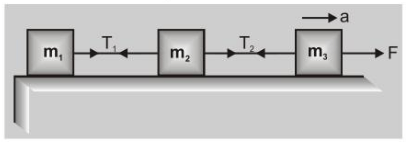Three bodies: In case of three bodies, the situation is shown in fig.

Acceleration $\quad a=\frac{F}{m_{1}+m_{2}+m_{3}}$ .....(4)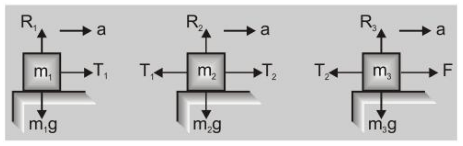$\mathrm{T}_{1}=\mathrm{m}_{1} \mathrm{a}=\frac{\mathrm{m}_{1} \mathrm{~F}}{\mathrm{~m}_{1}+\mathrm{m}_{2}+\mathrm{m}_{3}}$ ......(5)

$\mathrm{F}-\mathrm{T}_{2}=\mathrm{m}_{3} \mathrm{a}$

or$\mathrm{T}_{2}=\mathrm{F}-\frac{\mathrm{m}_{3} \mathrm{~F}}{\mathrm{~m}_{1}+\mathrm{m}_{2}+\mathrm{m}_{3}}=\frac{\left(\mathrm{m}_{1}+\mathrm{m}_{2}\right) \mathrm{F}}{\mathrm{m}_{1}+\mathrm{m}_{2}+\mathrm{m}_{3}}$    .....(6)

Ex. Three blocks, are connected by string as shown in fig. below, and are pulled by a force $T_{3}=120 \mathrm{~N}$. If $m_{1}=5 \mathrm{~kg}, m_{2}=10 \mathrm{~kg}$ and $m_{3}=15 \mathrm{~kg}$. Calculate the acceleration of the system and $T_{1}$ and $T_{2}$.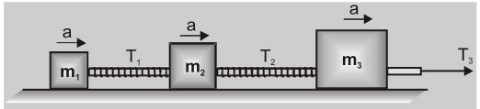Sol. (i) Acceleration of the system $\mathrm{a}=\frac{\mathrm{F}}{\mathrm{m}_{1}+\mathrm{m}_{2}+\mathrm{m}_{3}}=\frac{120}{5+10+15}=4 \mathrm{~m} / \mathrm{sec}^{2}$

(ii) $\mathrm{T}_{1}=\mathrm{m}_{1} \mathrm{a}=5 \times 4=20 \mathrm{~N}$           $T_{2}=\left(m_{1}+m_{2}\right) a=(5+10) 4=60 \mathrm{~N}$

Ex. A block of mass $M$ is pulled along a horizontal frictionless surface by a rope of mass $m$ as shown in fig. A horizontal force $F$ is applied to one end of the rope. Find (i) The acceleration of the rope and block (ii) The force that the rope exerts on the block. (iii) Tension in the rope at its mid point.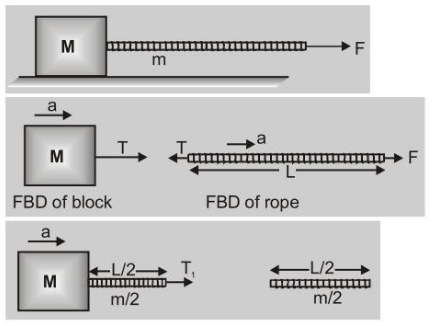Sol. (i) $F=(m+M) a \quad$ or $\quad a=\frac{F}{(m+M)}$

(ii) $\mathrm{T}=\mathrm{Ma}=\frac{\mathrm{M} \cdot \mathrm{F}}{(\mathrm{m}+\mathrm{M})}$

(iii) $\mathrm{T}_{1}=\left(\frac{\mathrm{m}}{2}+\mathrm{M}\right) \mathrm{a}=\left(\frac{\mathrm{m}+2 \mathrm{M}}{2}\right)\left(\frac{\mathrm{F}}{\mathrm{m}+\mathrm{M}}\right)$

or $\quad \mathrm{T}_{1}=\frac{(\mathrm{m}+2 \mathrm{M})}{2(\mathrm{~m}+\mathrm{M})} . \mathrm{F}$